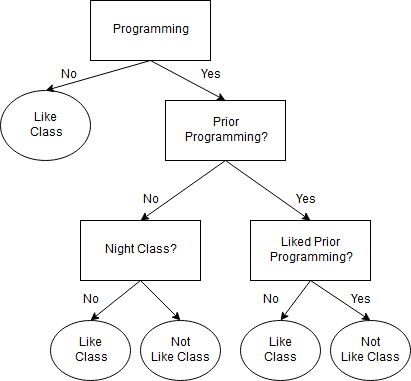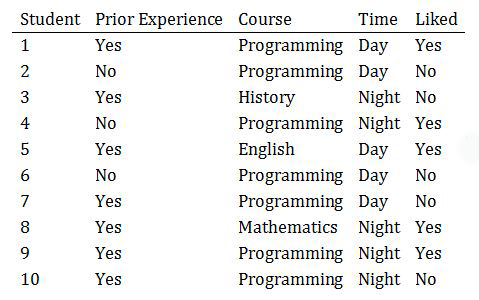Machine learning: decision trees revisited

By Jason M. Pittman, D.Sc.

Previously, we established learning as the ability to acquire knowledge. Further, I introduced the concept of decision trees as a basic model for machine learning. While I gave two examples of decision trees, one was abstract and the other simply illustrative. Those work for exploring the conceptual aspects but are clearly not enough if you want to get your hands dirty. Fortunately, decision trees are also a great introduction to programming machine learning algorithms. Let's dig in!

Decision tree algorithms

Decision tree algorithms come in two forms: classification and regression. The simplest way to conceptualize the difference is that classifiers are appropriate for binary decisions and regression is useful for predicting a relation between variables. Despite the difference in function and outcome, the algorithm structure is essentially the same. Moreover, algorithms don't select blindly. Generally, we have to train our decision tree algorithms using sample data (i.e., training datasets).

Let's use our programming class decision tree as an example again. Remember, we want to know if a given student will like a specific programming class.Further, let's assume that our training dataset looks something like the following. Maybe we have a friend in the registrar's office and he or she gave us a hand with gathering a sample of course history!We can implement an algorithm to construct the decision tree as follows. Note: we don't build a tree and then load training data. Instead, the tree is constructed from the training dataset.

1. Select the best attribute in the training dataset
2. Split data into a subset containing the values for our best attribute.
3. Encode the best attribute as a node.
4. Employ recursion to continue constructing nodes (using steps 2 and 3) from the leftover training data.

Seems straightforward, right? To be fair, there's more detail involved than what I'm letting on here. The beautiful thing is the details revolve around determining what is best. For example, in future posts we'll dive into cost (Gini) functions and entropy calculations. For now, we can let it stand that we have a classification algorithm capable of deciding if a student will like a programming course.

That aside, decision tree algorithms are not applicable to all problems. Decision trees don't generalize well, for one thing. Furthermore, decision tree algorithms tend to be greedy and, as a result, ignore global optimal solutions. Being greedy works well for deciding if a student will like a course but not so much for other situations. Furthermore, what we've outlined above is a supervised algorithm. Tune in next time when we push into more details and more types of algorithms!